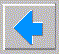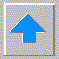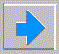# Lecture 12 - Probability and Final Philosophy (Last Lesson)

• Probability
• why do we need in AI ?
• insufficient knowledge (laziness?)
• hidden variables
• e.g. to differentiate between capital and small letters with no absolute size
• truly stochastic systems
• e.g. quantum mechanics, competing with humans
• interpretations of probability
1. relative frequency of repeating phenomenon
2. Bayesian interpretation (see below)
3. actual meaning in nature
4. subjective degree of belief
• example: what is the probability of the sun exploding today?
1. undefined since hasn't happened yet
2. zero since in all tests so far it hasn’t happened
3. p < 1/D where D is age of sun in days
4. determinable from statistics of other similar stars in universe
• axioms of probability theory
1. range 0 <= p <= 1
2. limits p(T) = 1 p(F) = 0
3. conditional probability p(A|B) = p(A And B) / p(B)
4. A and B are independent if p(A|B) = p(A) ie. p(A And B) = p(A) p(B)
• prove by p(A) + p(not A) = 1
5. disjunction p(A Or B) = p(A) + p(B) - p(A And B)
• mutually exclusive if P(A And B) = 0 i.e. p(A or B) = p(A) + p(B)
6. Bayes p(Hi|E) = p(E|Hi) p(Hi) / S i p(E|Hi) p(Hi)
• use to define probabilities without relative frequency
• Expert Systems that use probability
• if - then rules are replaced by conditional probabilities
• probabilities must be provided by the expert
• e.g. PROSPECTOR knows:
• prior probability for finding various minerals
• probability that if there is a given mineral then given evidence appears
• we need the probability - if evidence appears then mineral
• stock market expert example
• SR = stock market rises
• SF = stock market falls
• IR = interest rates rise
• IF = interest rates fall
• DR = dollar rises
• DF = dollar falls
• we want to know p(SR)
• p(SR) = p(SR|IR) p(IR) + p(SR|IF) p(IF)
• we need p(IR) (and then p(IF) = 1 - p(IR))
• p(IF) = p(IF|DR) p(DR) + P(IF|DF) p(DF)
• we need p(DR) (and then p(DF) = 1 - p(DR)) so we backtrack
• expert has preprogrammed values of p(X|Y)
• user must enter an estimate of p(DR) to get p(SR)
• Fuzzy Logic
• note the difference between:
• probabilistic case: 100 cups of which 90% coffee and 10% tea
• fuzzy case: 100 cups with 90% coffee and 10% tea in them
• fuzzy measure m(A)
• m(Not A) = 1 - m(A)
• m(A And B) = min( m(A), m(B) )
• m(A Or B) = max( m(A), m(B) )
• no need for normalization
• Final thoughts
• ethical questions
• it becomes clear that humans are not so special
• perhaps machines may be developed that will one day destroy humans
• legal questions
• if expert sustem passes Turing test will it deserve human rights?
• when physician uses a medical expert system, who is liable for malpractice?
• in US today ES is considered by courts to be like a textbook
• if ES provable to be better than MD, then perhaps he will be liable if doesn’t follow its advice
• if financial ES deals with stocks and bonds is owner liable for debts?
• can ES own assets and deal on its own behalf?
• ES car driver can legally exceed spped limit (but designer will be liable for damages)
• philosophical questions
• strong AI
• must a Turing test expert system be conscious ?
• brain prosthesis thought experiment
• replace neurons one at a time
• at end entire brain is artificial
• equivalently we could replace brain with a lookup table
• Have we really learned anything about AI ?
• Projects
• 1) any conversant program
• 2) any microworld agent
• 3) any artificial life program
• 4) any game playing program
• 5) any learning system
• 6) any expert system (car fault diag, internet searching, integration, from your work)
• 7) theorem prover (geometry, trig, word problems)
• 8) heuristic builder
• 9) test (default)